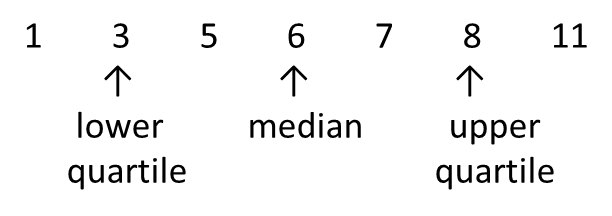# 7.2b Interquartile Range 1

Measures of Dispersion (Part 2)
7.2b Interquartile Range 1

(A) Interquartile Range of Ungrouped Data

Example 1:
Find the interquartile range of the following data.
(a) 7, 5, 1, 3, 6, 11, 8
(b) 12, 4, 6, 18, 9, 16, 2, 14

Solution:
(a) Rearrange the data according to ascending order.Interquartile Range
= upper quartile – lower quartile
= 8 – 3
= 5

(b) Rearrange the data according to ascending order.Interquartile Range
= upper quartile – lower quartile
$\frac{14+16}{2}-\frac{4+6}{2}$
= 15 – 5
= 10

(B) Interquartile Range of Grouped Data (without Class Interval)

Example 2:
The table below shows the marks obtained by a group of Form 4 students in school mid-term science test.

 Marks 1 2 3 4 5 Number of students 4 7 5 2 6

Determine the interquartile range of the distribution.

Solution:
Lower quartile, Q1 = the   $\frac{1}{4}{\left(24\right)}^{\text{th}}$ observation
= the 6thobservation
= 2

Upper quartile, Q3 = the   $\frac{3}{4}{\left(24\right)}^{\text{th}}$ observation
= the 18thobservation
= 4

 Marks Frequency Cumulative Frequency 1 4 4 2 7 11 (Q1 is located here) 3 5 16 4 2 18 (Q3 is located here) 5 6 24

Interquartile Range
= upper quartile – lower quartile
= Q3Q1
= 4 – 2
= 2